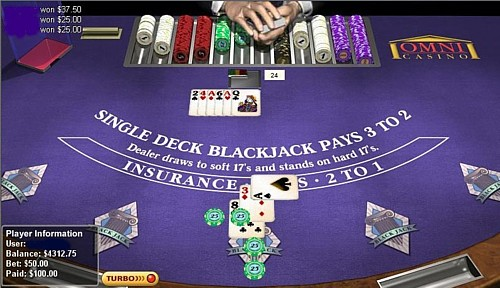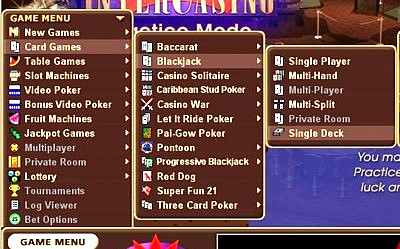Cryptologic single deck blackjack

• House edge and rules
• Basic strategy charts
• Unusual plays
• Recommended casinos
• Game locationHouse edge and rules

Cryptologic currently have a single-deck blackjack game with an overall payout of 99.59% and a house edge of 0.41%.

The rules are:

• Hard 17
• Double on 9, 10 and 11 only
• No Double After Split
• No peek - (ENHC)

These are very poor rules, which give this single deck game a return worse than some eight deckers.

Basic strategy charts

The following is the correct basic strategy for this game - the top row represents the dealer's up card, the left-hand column the player hand:

S = stand, H = hit, D = double, P = split

 2 3 4 5 6 7 8 9 10 A 17 S S S S S S S S S S 16 S S S S S H H H H H 15 S S S S S H H H H H 14 S S S S S H H H H H 13 S S S S S H H H H H 12 H H S S S H H H H H 11 D D D D D D D D H H 10 D D D D D D D D H H 9 D D D D D H H H H H 8 H H H H H H H H H H

 2 3 4 5 6 7 8 9 10 A A8 S S S S S S S S S S A7 S S S S S S S H H S A6 H H H H H H H H H H A5 H H H H H H H H H H A4 H H H H H H H H H H A3 H H H H H H H H H H A2 H H H H H H H H H H

 2 3 4 5 6 7 8 9 10 A As P P P P P P P P P H 10s S S S S S S S S S S 9s P P P P P S P P S S 8s P P P P P P P P H H 7s P P P P P P H H S H 6s P P P P P H H H H H 5s D D D D D D D D H H 4s H H H H H H H H H H 3s H H P P P P H H H H 2s H P P P P P H H H H

Unusual plays

There are two slightly unusual plays here, particularly if you're used to the standard multi-deck strategy. They are:

9 v. 2 = double, not hit.
77 v. 10 = stand, not hit.

Recommended casinos

The casino I recommend is:

♠ InterCasino ♠

Game location

For the location of the game see the screenshot below:Ensure that you have the single deck game correctly selected - it sits right at the bottom of the blackjack menu in each of the casinos.

Page top

© 2005 - 2021 Hundred Percent Gambling